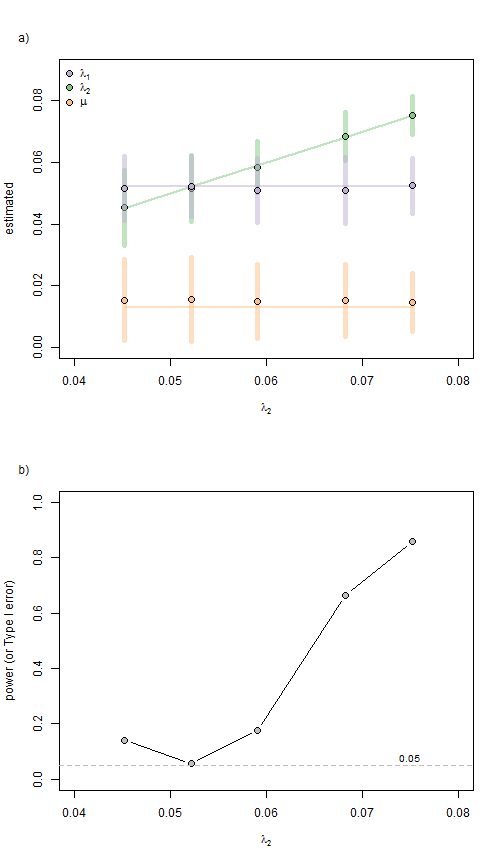## Monday, November 6, 2017

### Parameter estimation and power in `ratebytree` method to compare speciation & extinction between trees

I recently added to the function `ratebytree` methods to compare the rates of diversification (speciation, extinction, or both) between phylogenies (1, 2, 3, 4, 5).

In the following I'm going to look at parameter estimation & statistical power of the method. For this simulation I generated pairs of phylogenies in which I held λ1 constant at 0.052, μ constant at 0.013, the total time T constant at 100 (this results in an expected number of lineages equal to 100 in tree 1), and then varied λ2 to be equal to 0.045, 0.052, 0.059, 0.068, and 0.075 to result in an expected number of extant lineages of 50, 100, 200, 500, and 1000 (respectively) in tree 2. Finally, I fit the generating model (a variable speciation model) to each pair of trees.

Note that I repeated any simulation that resulted in a tree containing fewer than five taxa.

Here is what that looked like:

``````library(phytools)
set.seed(1)
## general
N<-c(50,100,200,500,1000)
T<-100
nd<-(log(N)-log(2))/T
b1<-nd/(1-0.25)
d<-b1-nd
b2<-(log(N)-log(2))/T+d
nsim<-500

## our birth-death simulator
foo<-function(b1,b2,...){
t1<-NULL
while(is.null(t1)||Ntip(t1)<5) t1<-pbtree(b=b1,...,quiet=T)
t2<-NULL
while(is.null(t2)||Ntip(t2)<5) t2<-pbtree(b=b2,...,quiet=T)
obj<-c(t1,t2)
class(obj)<-"multiPhylo"
obj
}

## some objects to use to store our results
FITS<-list()
RESULTS<-list()
BD<-matrix(NA,length(b2),7,dimnames=list(paste("sim",1:length(b2)),
c("b","sd(b)","b","sd(b)","d","sd(d)","power")))

for(i in 1:length(b2)){
t<-replicate(nsim,foo(b1=b1,b2=b2[i],d=d,t=T,extant.only=TRUE),
simplify=FALSE)
fits<-lapply(t,ratebytree,model="equal-extinction")
RESULTS[[i]]<-cbind(
sapply(fits,function(x) x\$multi.rate.model\$rates),
sapply(fits,function(x) x\$multi.rate.model\$rates),
sapply(fits,function(x) x\$multi.rate.model\$rates),
sapply(fits,function(x) x\$likelihood.ratio),
sapply(fits,function(x) x\$P.chisq))
colnames(RESULTS[[i]])<-c("b","b","d","LR","P")
FITS[[i]]<-fits
BD[i,c(1,3,5)]<-colMeans(RESULTS[[i]][,1:3])
BD[i,c(2,4,6)]<-apply(RESULTS[[i]][,1:3],2,sd)
BD[i,7]<-mean(RESULTS[[i]][,5]<=0.05)
}
``````
``````## There were 50 or more warnings (use warnings() to see the first 50)
``````
``````## RESULTS
print(round(BD,5))
``````
``````##          b sd(b)    b sd(b)       d   sd(d) power
## sim 1 0.05161  0.01051 0.04537  0.01217 0.01537 0.01316 0.140
## sim 2 0.05216  0.00968 0.05162  0.01075 0.01554 0.01375 0.058
## sim 3 0.05081  0.01040 0.05853  0.00838 0.01483 0.01201 0.178
## sim 4 0.05100  0.01073 0.06842  0.00786 0.01518 0.01162 0.664
## sim 5 0.05241  0.00887 0.07525  0.00618 0.01469 0.00945 0.858
``````
``````par(mfrow=c(2,1))

library(RColorBrewer)
cols<-brewer.pal(3,"Accent")
xlim<-c(0.04,0.08)
ylim<-c(0,0.09)

plot(b2,BD[,3],xlim=xlim,ylim=ylim,pch=21,bg=cols,cex=1.25,
xlab=expression(lambda),ylab="estimated")
lines(b2,b2,lwd=2,col=make.transparent(cols,0.5))
for(i in 1:length(b2))
lines(rep(b2[i],2),rep(BD[i,3])+c(BD[i,4],-BD[i,4]),lwd=5,
col=make.transparent(cols,0.5))
points(b2,BD[,3],pch=21,cex=1.25)

points(b2,BD[,1],pch=21,bg=cols,cex=1.25)
lines(b2,rep(b1,length(b2)),lwd=2,col=make.transparent(cols,0.5))
for(i in 1:length(b2))
lines(rep(b2[i],2),rep(BD[i,1])+c(BD[i,2],-BD[i,2]),lwd=5,
col=make.transparent(cols,0.5))
points(b2,BD[,1],pch=21,cex=1.25)

points(b2,BD[,5],pch=21,bg=cols,cex=1.25)
lines(b2,rep(d,length(b2)),lwd=2,col=make.transparent(cols,0.5))
for(i in 1:length(b2))
lines(rep(b2[i],2),rep(BD[i,5])+c(BD[i,6],-BD[i,6]),lwd=5,
col=make.transparent(cols,0.5))
points(b2,BD[,5],pch=21,cex=1.25)

legend(x=par()\$usr,y=par()\$usr,
legend=c(expression(lambda),expression(lambda),
expression(mu)),pch=21,pt.bg=cols[c(2,1,3)],pt.cex=1.25,bty="n")

plot(b2,BD[,7],xlim=xlim,ylim=c(0,1),pch=21,bg="grey",type="b",cex=1.25,
xlab=expression(lambda),ylab="power (or Type I error)")
abline(h=0.05,lty="dashed",col="grey")
text(x=0.075,y=0.04,"0.05",pos=3,cex=0.8)

``````In panel a), the generating values of the parameters are denoted by the various color diagonal or horizontal lines, while the mean estimates (and SD across simulations) are given by the points and vertical lines, respectively.

In panel b), the power (or type I error in the case of λ1 = λ2) are given by the points. The horizontal dotted line is at the nominal α level (α = 0.05).

Neat.